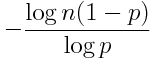# Probability of long runs

Suppose you’ve written a program that randomly assigns test subjects to one of two treatments, A or B, with equal probability. The researcher using your program calls you to tell you that your software is broken because it has assigned treatment A to seven subjects in a row.

You might argue that the probability of seven A’s in a row is (1/2)7 or about 0.008. Not impossible, but pretty small. Maybe the software is broken.

But this line of reasoning grossly underestimates the probability of a run of 7 identical assignments. If someone asked the probability that the next 7 assignments would all be A’s, then (1/2)7 would be the right answer. But that’s not the same as asking whether an experiment is likely to see a run of length 7 because the run could start any time, not just on the next assignment. Also, the phone didn’t ring out of the blue: it rang precisely because there had just been a run.

Suppose you have a coin that has probability of heads p and you flip this coin n times. A rule of thumb says that the expected length of the longest run of heads is aboutprovided that n(1-p) is much larger than 1.

So in a trial of n = 200 subjects with p = 0.5, you’d expect the longest run of heads to be about seven in a row. When p is larger than 0.5, the longest expected run will be longer. For example, if p = 0.6, you’d expect a run of about 9.

The standard deviation of the longest run length is roughly 1/log(1/p), independent of n. For coin flips with equal probability of heads or tails, this says an approximate 95% confidence interval would be about 3 either side of the point estimate. So for 200 tosses of a fair coin, you’d expect the longest run of heads to be about 7 ± 3, or between 4 and 10.

The following Python code gives an estimate of the probability that the longest run is between a and b inclusive, based on an extreme value distribution.

```def prob(a, b, n, p):
r = -log(n*(1-p))/log(p)
cdf = lambda x: exp(- p**x )
return cdf(b + 1 - r) - cdf(a - r)```

What if you were interested in the longest run of head or tails? With a fair coin, this just adds 1 to the estimates above. To see this, consider a success to be when consecutive coins turn up the same way. This new sequence has the same expected run lengths, but a run of length m in this sequence corresponds to a run of length m + 1 in the original sequence.

For more details, see “The Surprising Predictability of Long Runs” by Mark F. Schilling, Mathematics Magazine 85 (2012), number 2, pages 141–149.

RelatedNeed help with randomization?

## 10 thoughts on “Probability of long runs”

1. A few years ago, Radiolab had an episode called Stochasticity, in which they talked about the likelihood of seemingly unlikely events. One of the examples was a run of seven tails in sequence of 100 coin flips. I wrote a post about how to calculate the probability of such runs, which involves k-step Fibonacci numbers, but never considered the statistics of the longest run. It’s fun to see a different (and, frankly, much simpler) way of looking at the problem.

2. Luis Mendo

Could you provide a reference for the expression of the expected length of the longest run? I would like to read more on that. Thanks!

3. Luis: Please see the paper by Mark F. Schilling in the last line of the post.

4. Luis Mendo

Whoops, I didn’t see that! Thanks

5. A Confused One

My python says `1/m.log10(1/0.5) 3.321928094887362`, but `math.log` means ln. So what’s the base of your log? 10, e, 2?

6. When I write “log,” I always mean natural log.

7. A Confused One

Thanks you for your explanation – and the follow-up post :).

8. ezra abrams

thanks
if there were a collection of 100 surprising math facts about the real world every scientists and engineer should know, this would surely be on the list.

9. Douglas Zare

Hi. Someone linked to this post from http://mathoverflow.net/questions/125814/maximal-chain-of-1s-in-binary-strings. I have a few comments.

In the motivating statistical experiment, it may make sense to choose the assignments in a more balanced fashion than assigning individuals independently. For example, if there are 10 subjects, you might choose randomly from among the ways to assign 5 to each treatment rather than risking the assignment of 9/10 to treatment A by luck.

The formula you give for the standard deviation differs from the one in Schilling’s papers by a factor of pi/sqrt(6).

The distribution about the mean is not normal. The tails drop off only roughly exponentially (for a while).

10. John Borneman

Is there a way to predict runs when p is very low? i.e. below 0.01 or less?